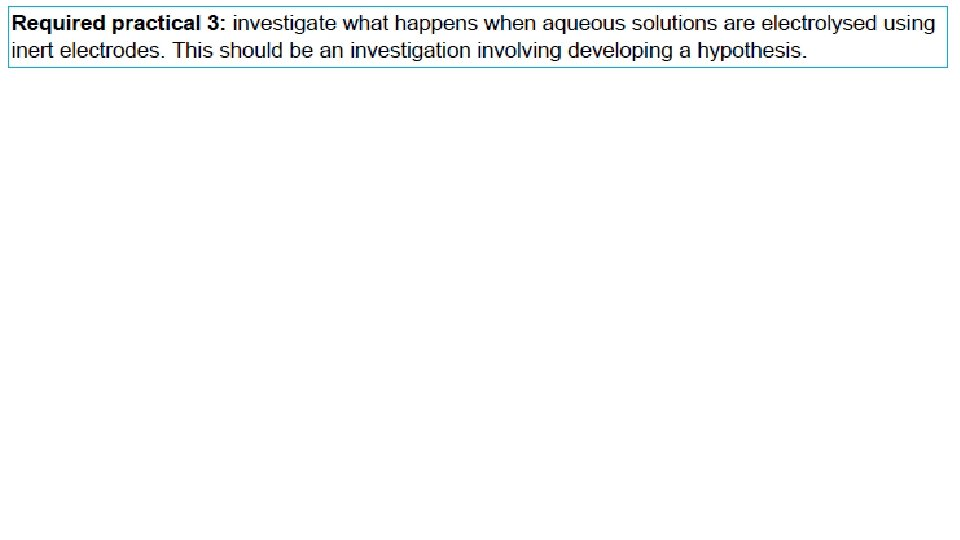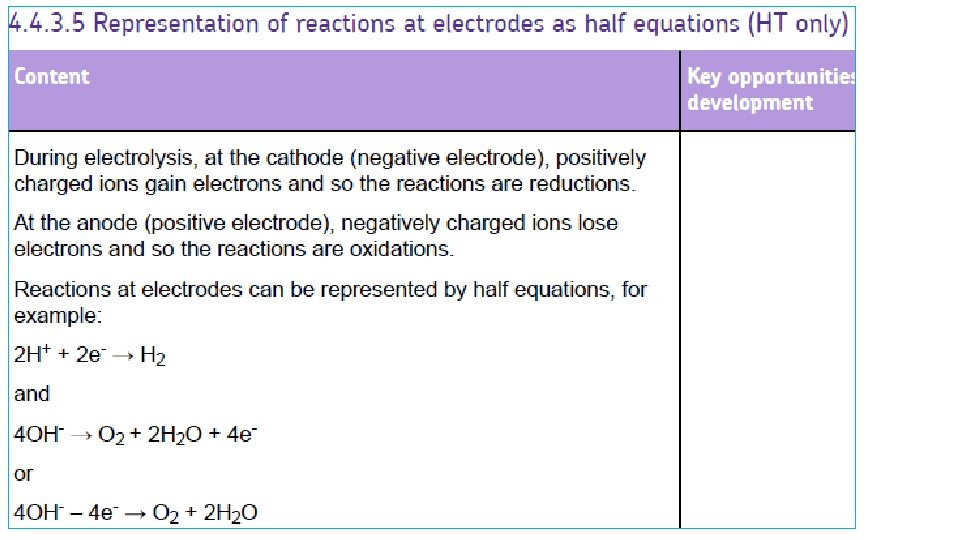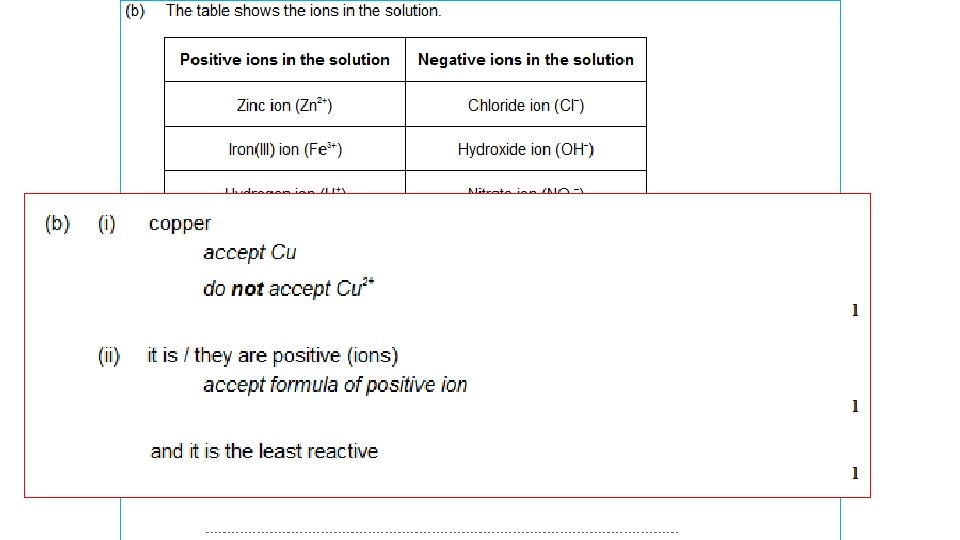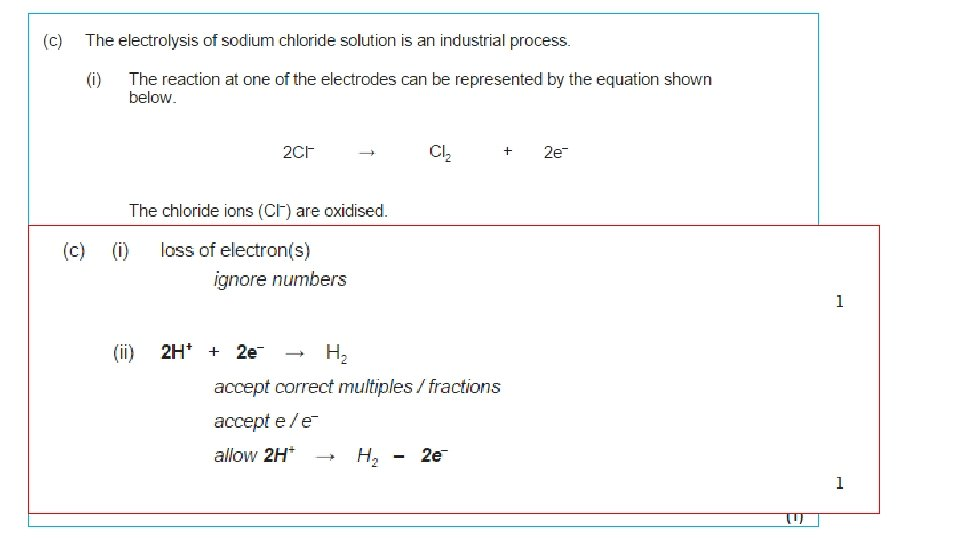Oxidation Magnesium oxygen Magnesium oxide Magnesium is OXIDISED

• Slides: 72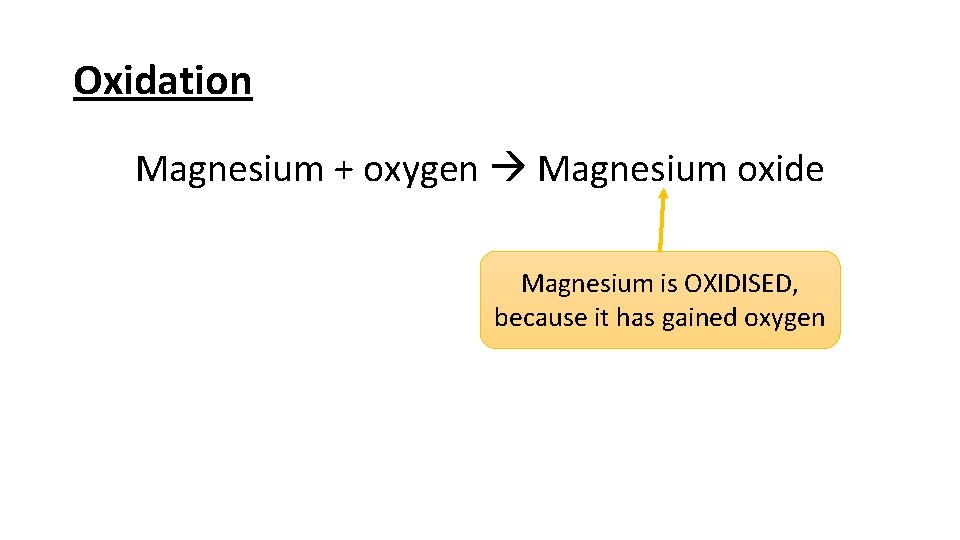Oxidation Magnesium + oxygen Magnesium oxide Magnesium is OXIDISED, because it has gained oxygen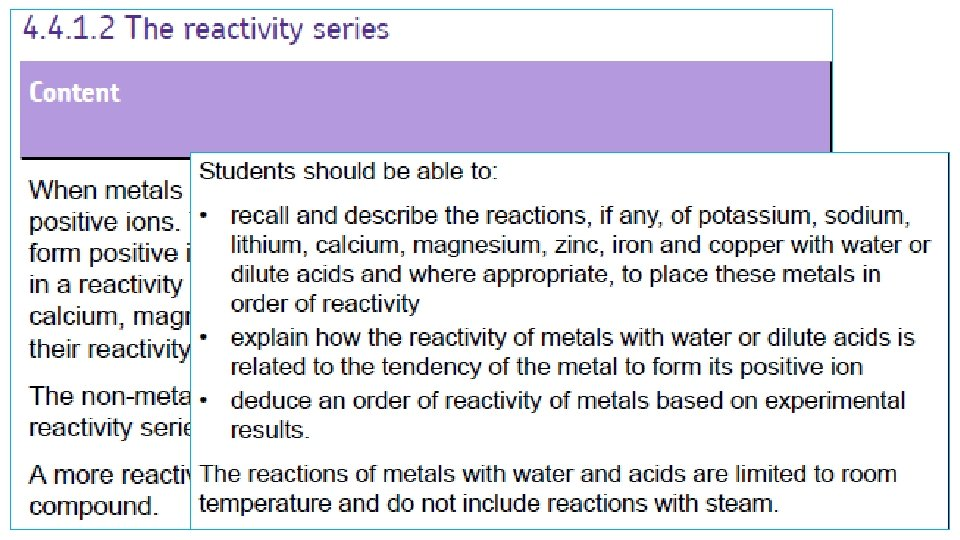Reactivity series Potassium Sodium Lithium Calcium Magnesium Carbon Zinc Iron Hydrogen Copper Silver Gold ELECTROLYSIS REDUCTION NATIVE METALS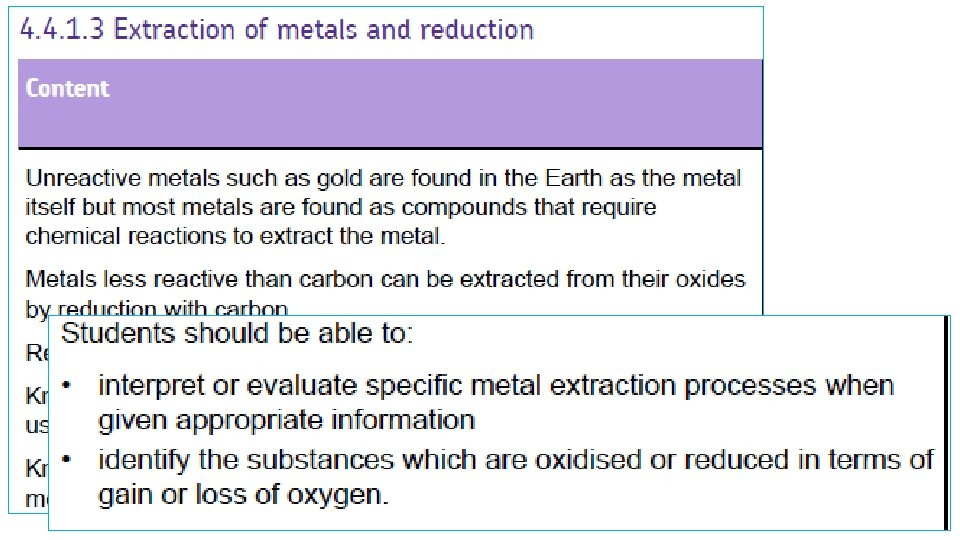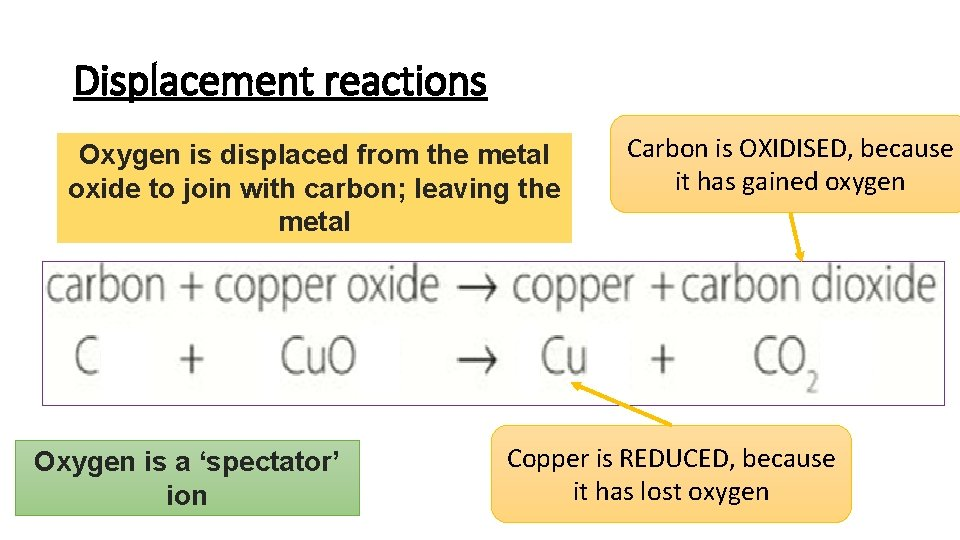Displacement reactions Oxygen is displaced from the metal oxide to join with carbon; leaving the metal Oxygen is a ‘spectator’ ion Carbon is OXIDISED, because it has gained oxygen Copper is REDUCED, because it has lost oxygen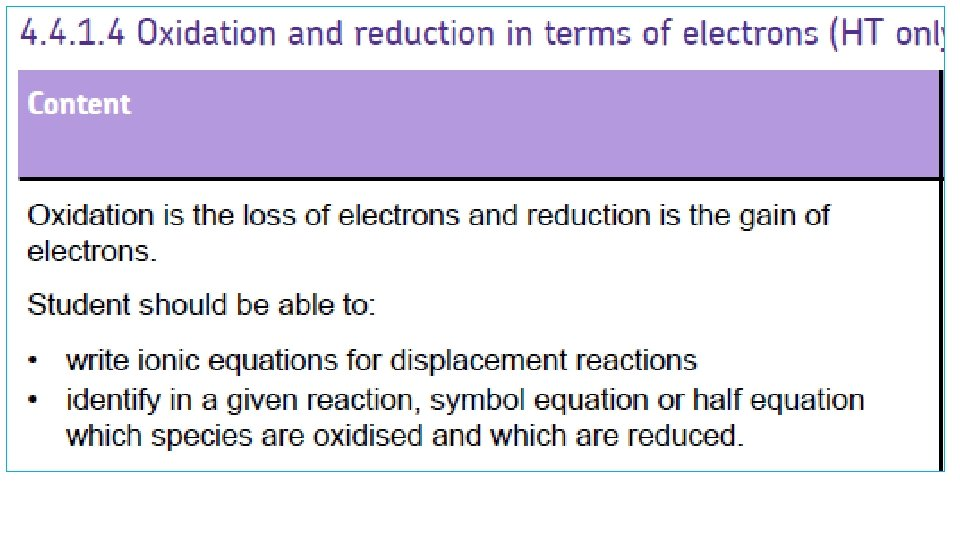Reduction and oxidation reactions Fe 2 O 3 + Al Fe + Al 2 O 3 Fe 3+ Al 0 Fe 0 Al 3+ Fe 3+ + 3 e- Fe Reduction Al 2 O 3 + 3 e- Oxidation Oxygen is a ‘spectator’ ion Ionic half-equationsOIL RIG OXIDATION is LOSS (of electrons) REDUCTION is GAIN (of electrons)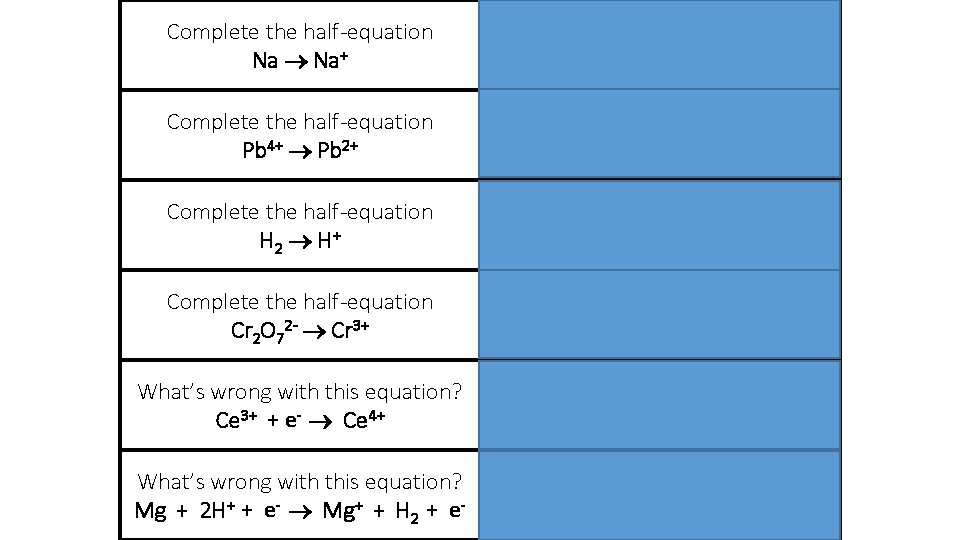Complete the half-equation Na Na++ e- Complete the half-equation Pb 4+ Pb 2+ Pb 4+ + 2 e- Pb 2+ Complete the half-equation H 2 H + H 2 2 H+ + 2 e- Complete the half-equation Cr 2 O 72 - Cr 3+ Cr 2 O 72 - + 6 e- 2 Cr 3+ What’s wrong with this equation? Ce 3+ + e- Ce 4+ Electron is on the wrong side! What’s wrong with this equation? Mg + 2 H+ + e- Mg+ + H 2 + e- Should be Mg 2+ Electrons should be cancelled out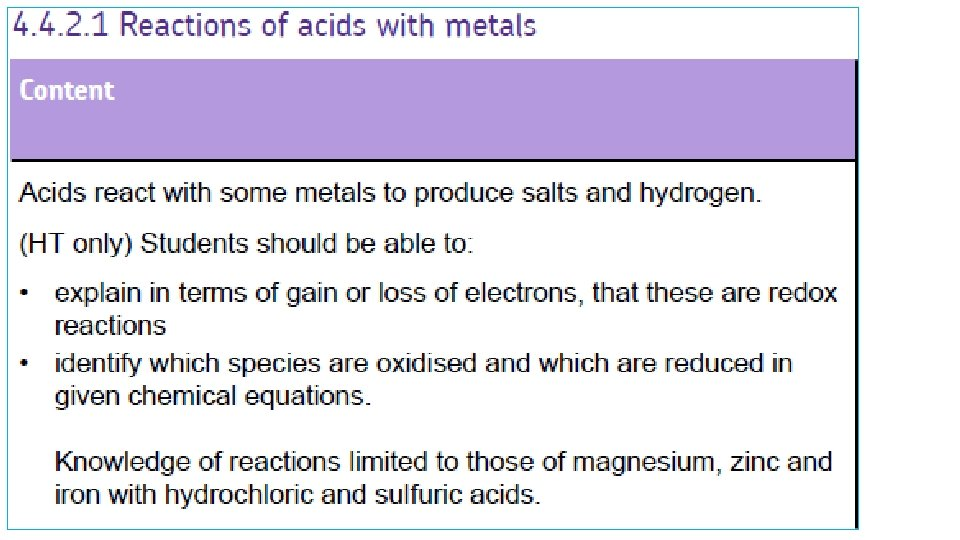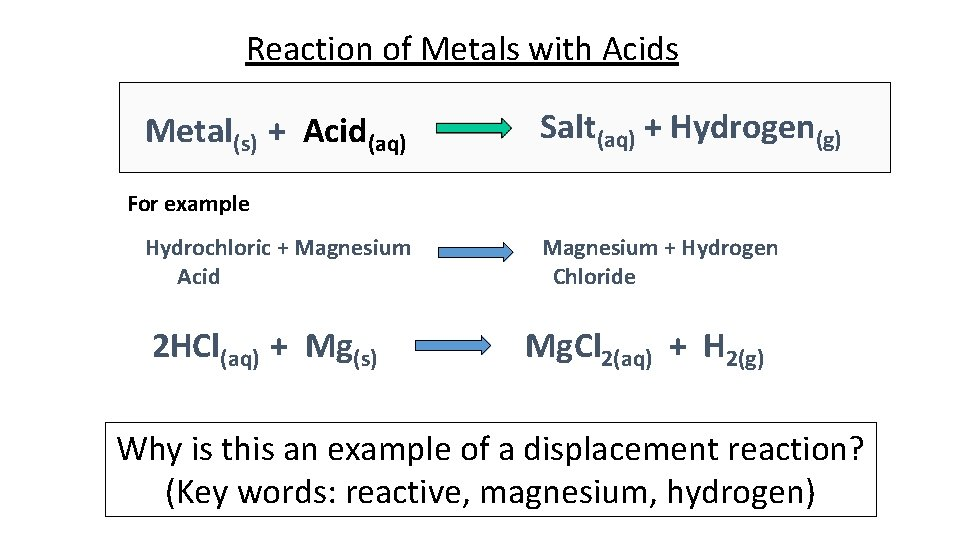Reaction of Metals with Acids Metal(s) + Acid(aq) Salt(aq) + Hydrogen(g) For example Hydrochloric + Magnesium Acid 2 HCl(aq) + Mg(s) Magnesium + Hydrogen Chloride Mg. Cl 2(aq) + H 2(g) Why is this an example of a displacement reaction? (Key words: reactive, magnesium, hydrogen)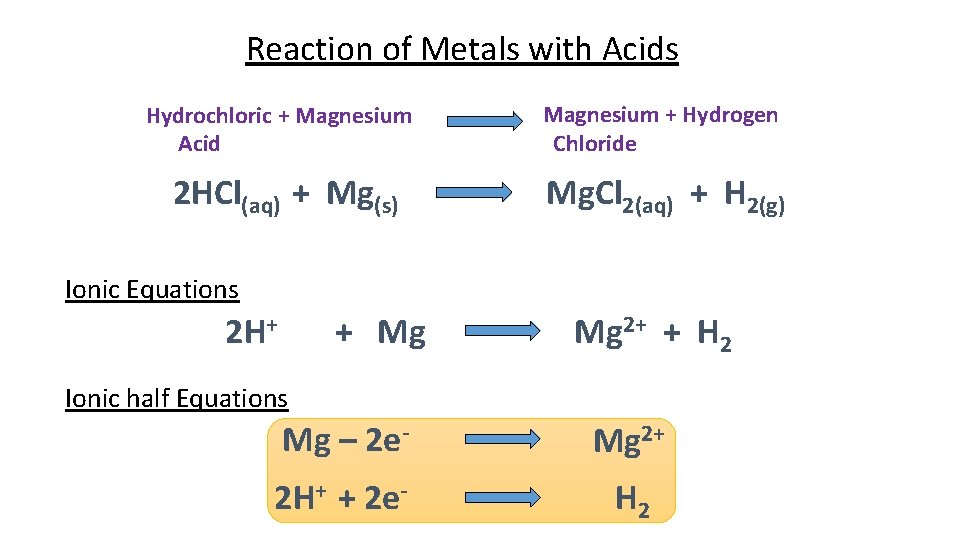Reaction of Metals with Acids Hydrochloric + Magnesium Acid Magnesium + Hydrogen Chloride 2 HCl(aq) + Mg(s) Mg. Cl 2(aq) + H 2(g) Ionic Equations 2 H+ + Mg Mg 2+ + H 2 Ionic half Equations Mg – 2 e- Mg 2+ 2 H+ + 2 e- H 2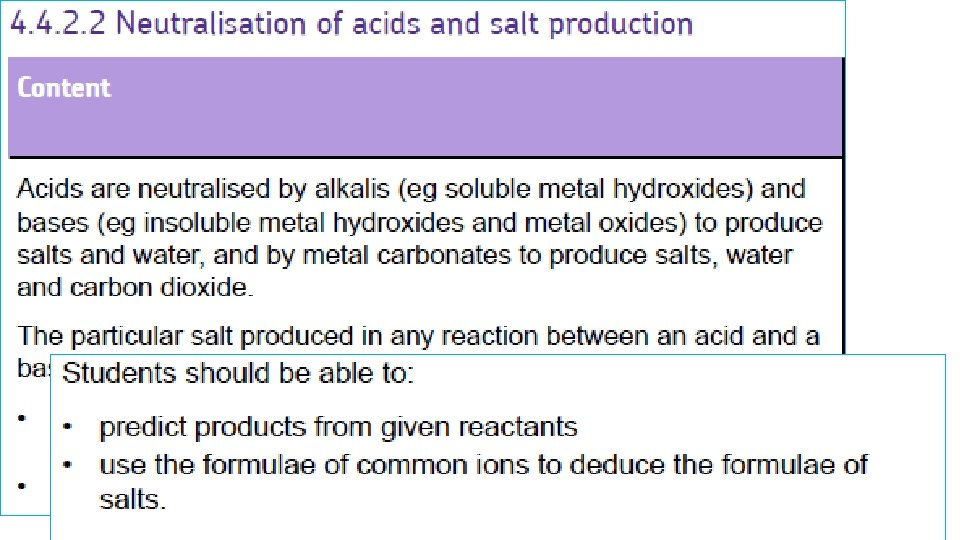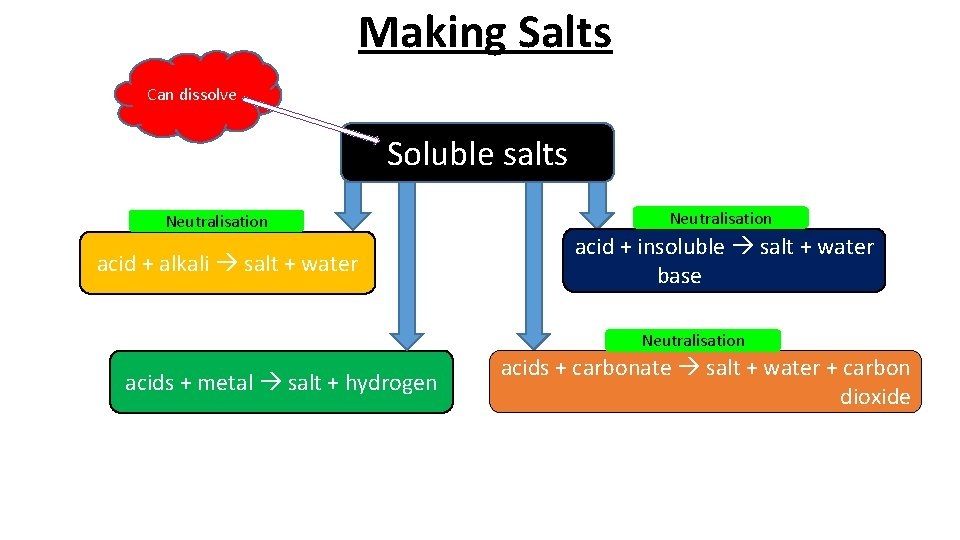Making Salts Can dissolve Soluble salts Neutralisation acid + alkali salt + water Neutralisation acid + insoluble salt + water base Neutralisation acids + metal salt + hydrogen acids + carbonate salt + water + carbon dioxideWhat salt is produce metal hydrochloric sulfuric nitric acid metal salt chloride sulfate nitrate hydrogen The salt produced depends on the metal (or metal compound) and type of acid involved in the reaction: l When a metal reacts with hydrochloric acid, the salt produced is a metal chloride. l When a metal reacts with sulfuric acid, the salt produced is a metal sulfate. l When a metal reacts with nitric acid, the salt produced is a metal nitrate.Complete the word equations Complete the word & symbol equations for metals reacting with acid: magnesium nitric acid magnesium ? nitrate hydrogen Symbol: iron sulfuric acid iron ? sulfate hydrogen hydrochloric acid zinc ? chloride sulfuric ? acid lead sulfate hydrogen Symbol: lead ? Symbol: Acids Nitric acid = HNO 3 Sulfuric acid = H 2 SO 4 Hydrocholric acid = HCl Symbol: zinc What element do the acids have in common? hydrogen Salts Nitrate = NO 3 Sulfate = SO 4 Chloride = ClReaction of Bases with Acids Neutralisation Acid(aq) + Base(s) Salt(aq) + Water(l) For example 6 HCl(aq) + Fe 2 O 3(s) Hydrochloric + Iron (III) Acid Oxide 2 Fe. Cl 3(aq) + 3 H 2 O(l) Iron (III) + Water Chloride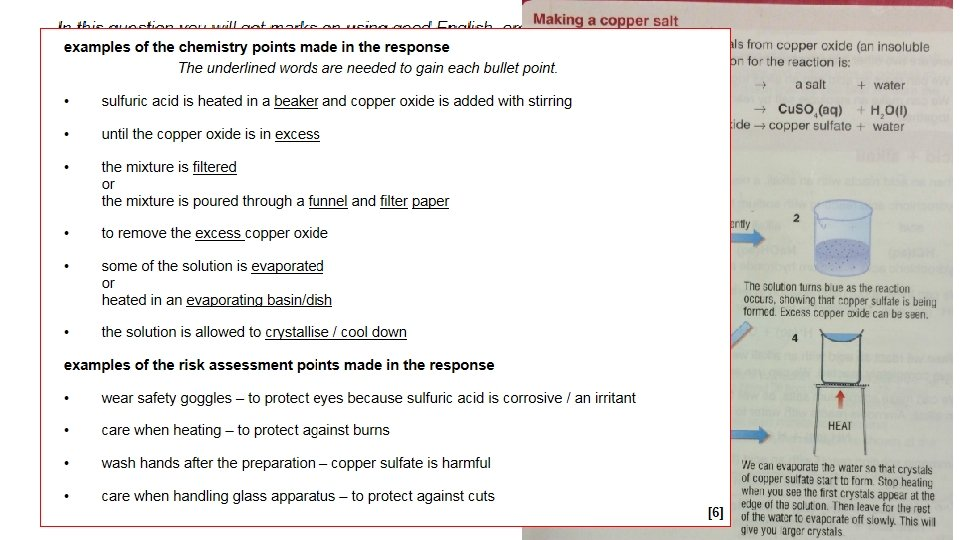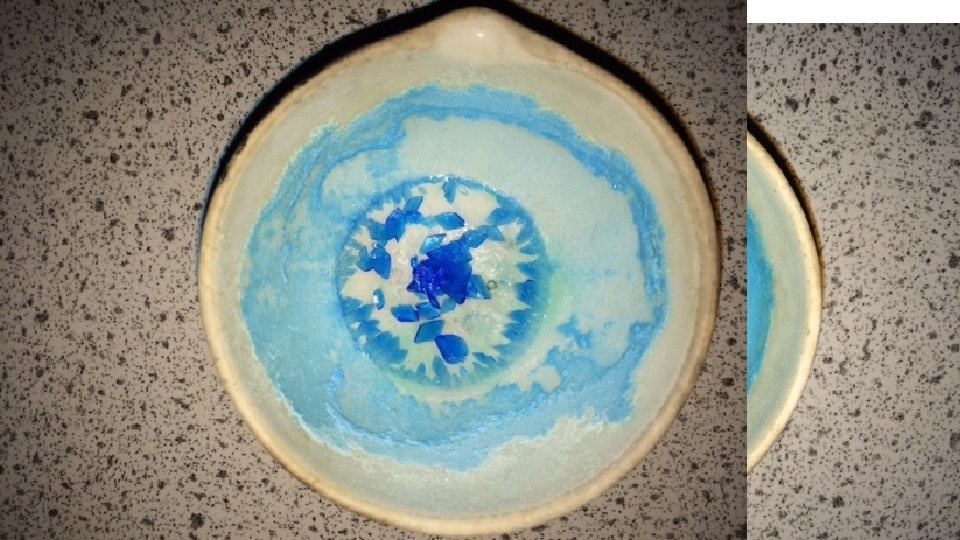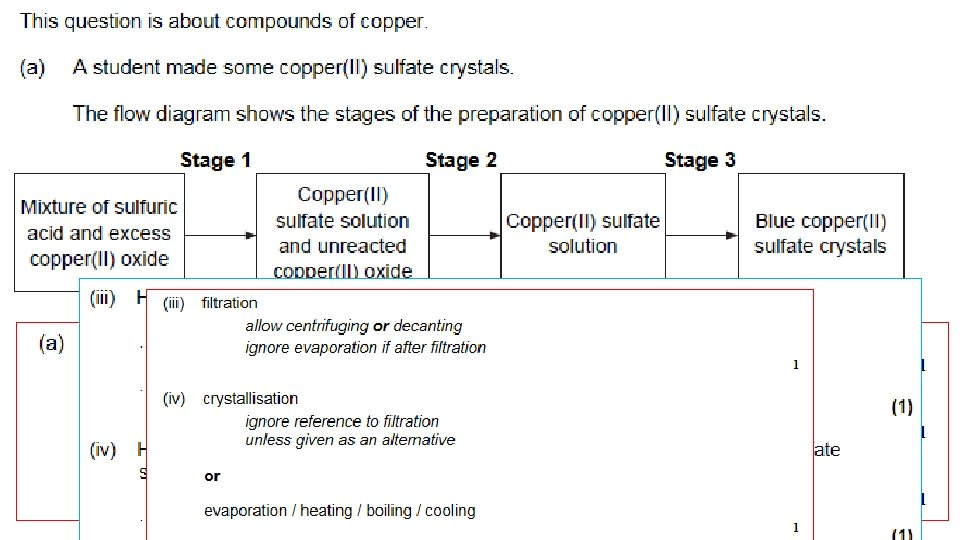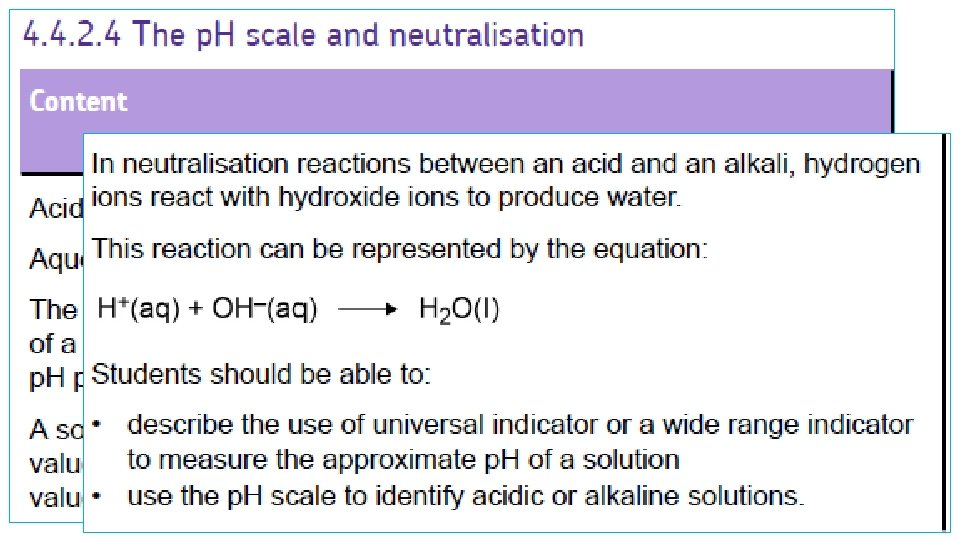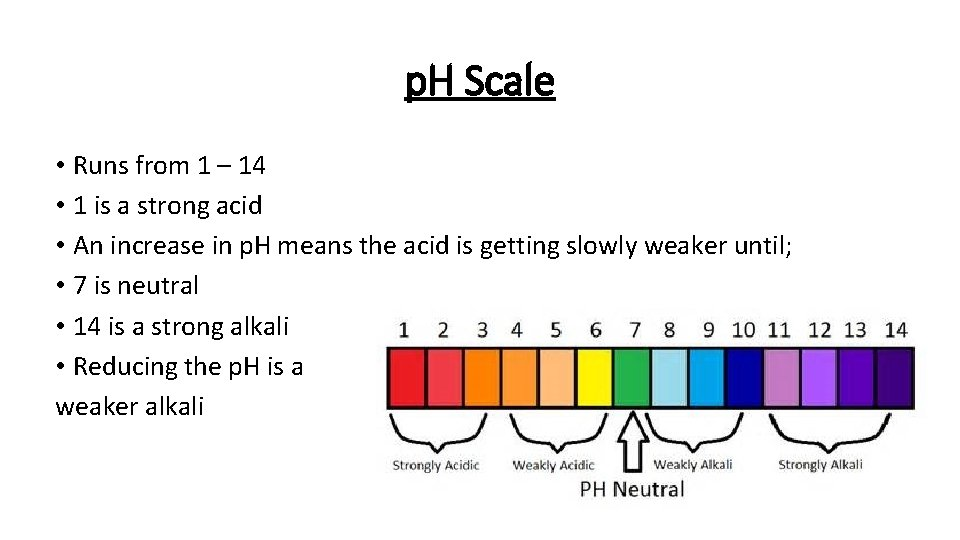p. H Scale • Runs from 1 – 14 • 1 is a strong acid • An increase in p. H means the acid is getting slowly weaker until; • 7 is neutral • 14 is a strong alkali • Reducing the p. H is a weaker alkaliAcids • These are substances that contain hydrogen ions (H+) • The p. H scale is a measure of the amount of H+ a solution contains. • Low p. H means high H+ and high acidityBases • These are substances which can neutralise acids. Metal oxides and hydroxides (eg magnesium oxide and magnesium hydroxide) are bases. Substance with a p. H GREATER than 7 • Bases that dissolve in water are called alkalis and produce OH- (hydroxide ions) in solution. BASE • Bases and alkalis have a high p. H over 7 on the p. H scale. Dissolves in water ALKALI(aq)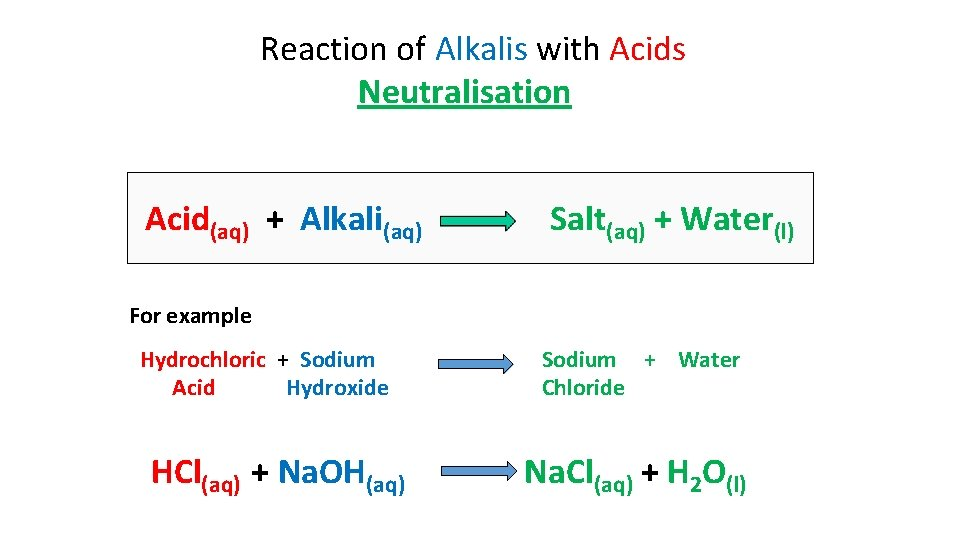Reaction of Alkalis with Acids Neutralisation Acid(aq) + Alkali(aq) Salt(aq) + Water(l) For example Hydrochloric + Sodium Acid Hydroxide HCl(aq) + Na. OH(aq) Sodium + Water Chloride Na. Cl(aq) + H 2 O(l)Creating a salt from an acid and alkali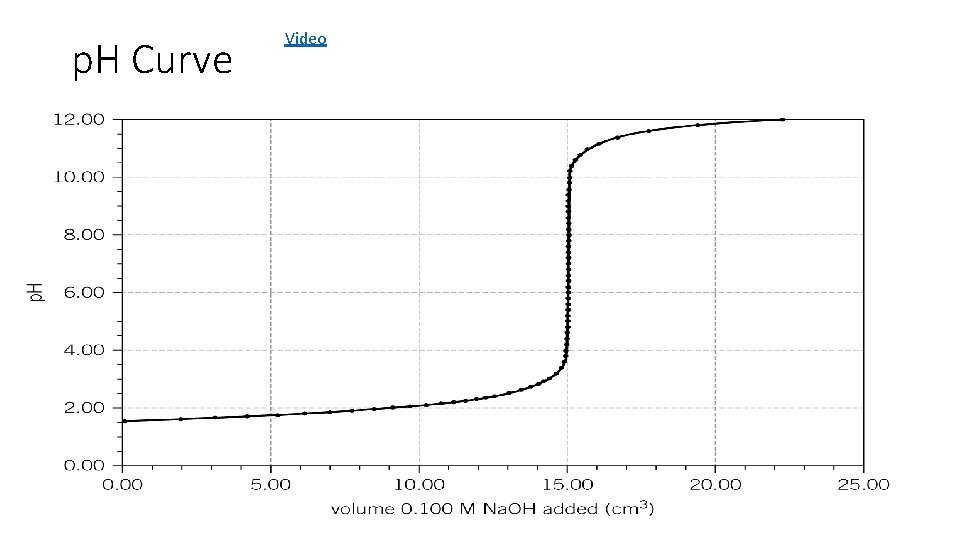p. H Curve Video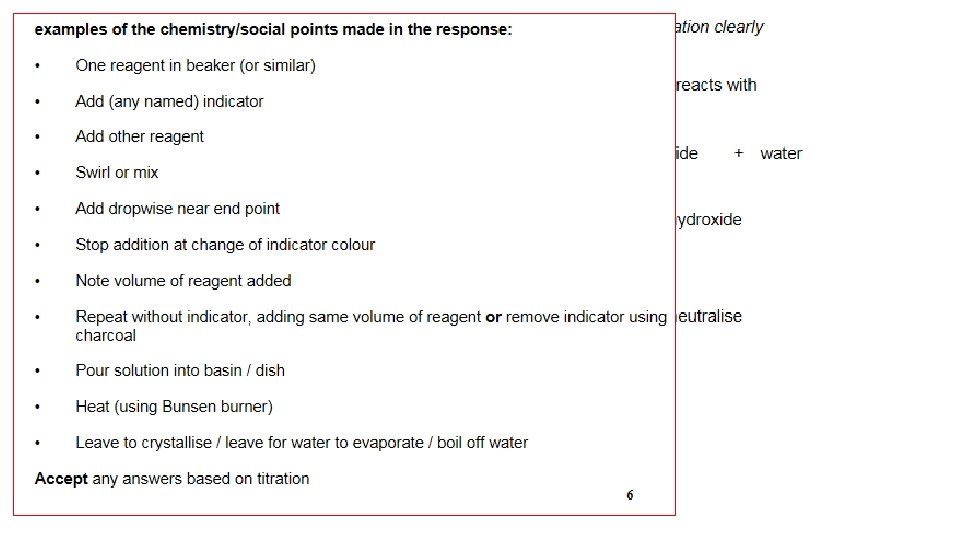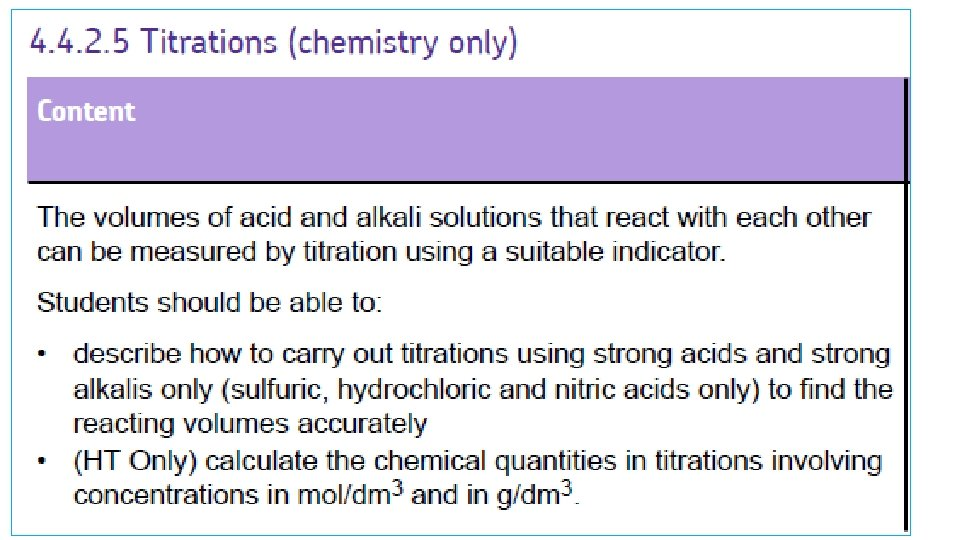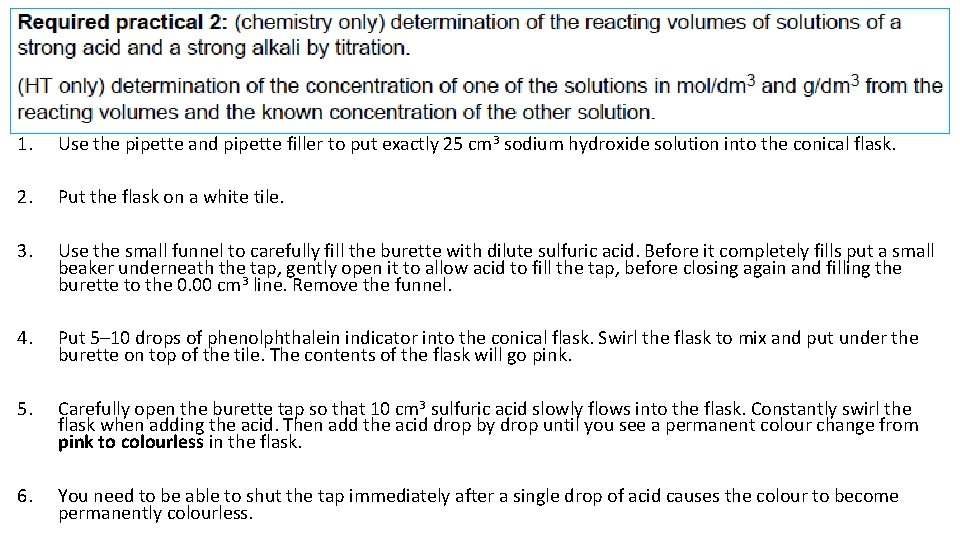1. Use the pipette and pipette filler to put exactly 25 cm 3 sodium hydroxide solution into the conical flask. 2. Put the flask on a white tile. 3. Use the small funnel to carefully fill the burette with dilute sulfuric acid. Before it completely fills put a small beaker underneath the tap, gently open it to allow acid to fill the tap, before closing again and filling the burette to the 0. 00 cm 3 line. Remove the funnel. 4. Put 5– 10 drops of phenolphthalein indicator into the conical flask. Swirl the flask to mix and put under the burette on top of the tile. The contents of the flask will go pink. 5. Carefully open the burette tap so that 10 cm 3 sulfuric acid slowly flows into the flask. Constantly swirl the flask when adding the acid. Then add the acid drop by drop until you see a permanent colour change from pink to colourless in the flask. 6. You need to be able to shut the tap immediately after a single drop of acid causes the colour to become permanently colourless.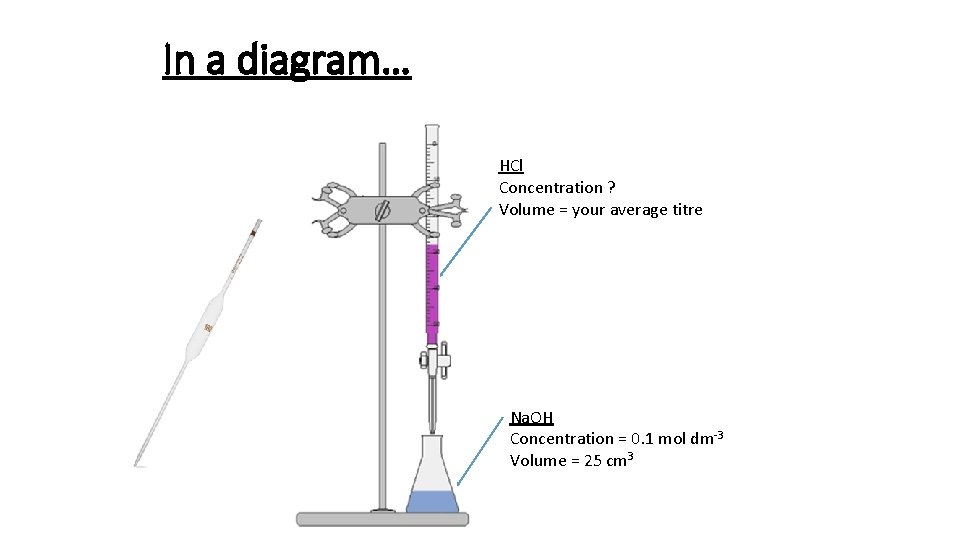In a diagram… HCl Concentration ? Volume = your average titre Na. OH Concentration = 0. 1 mol dm-3 Volume = 25 cm 3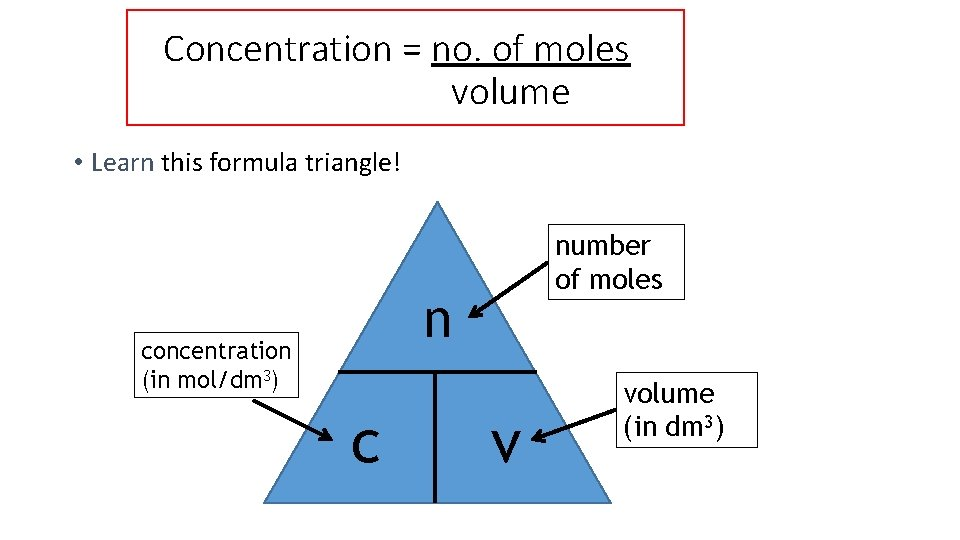Concentration = no. of moles volume • Learn this formula triangle! number of moles n concentration (in mol/dm 3) c v volume (in dm 3)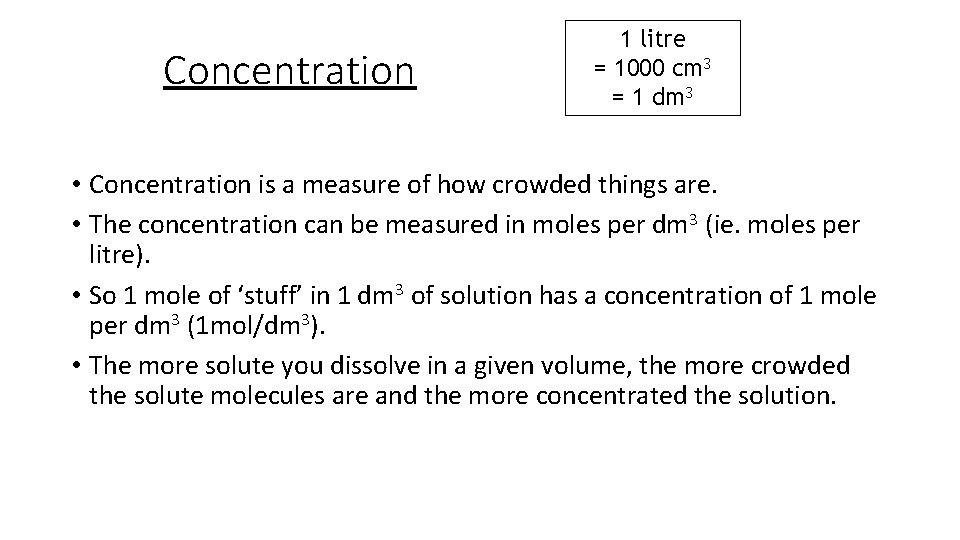Concentration 1 litre = 1000 cm 3 = 1 dm 3 • Concentration is a measure of how crowded things are. • The concentration can be measured in moles per dm 3 (ie. moles per litre). • So 1 mole of ‘stuff’ in 1 dm 3 of solution has a concentration of 1 mole per dm 3 (1 mol/dm 3). • The more solute you dissolve in a given volume, the more crowded the solute molecules are and the more concentrated the solution.Using the triangle • Example 1: What is the concentration of a solution with 2 moles of salt in 500 cm 3? The question already tells us the number of moles and the volume, so use the formula: c = n = 2 = 4 mol/dm 3 convert the v 0. 5 volume to dm 3 first by dividing by 1000.Using the triangle • Example 2: How many moles of sodium chloride are in 250 cm 3 of a 3 mol/dm 3 solution of sodium chloride? The question tells us the volume and concentration, so use the formula: n = c x v = 3 x 0. 25 = 0. 75 moles convert the volume to dm 3 first by dividing by 1000.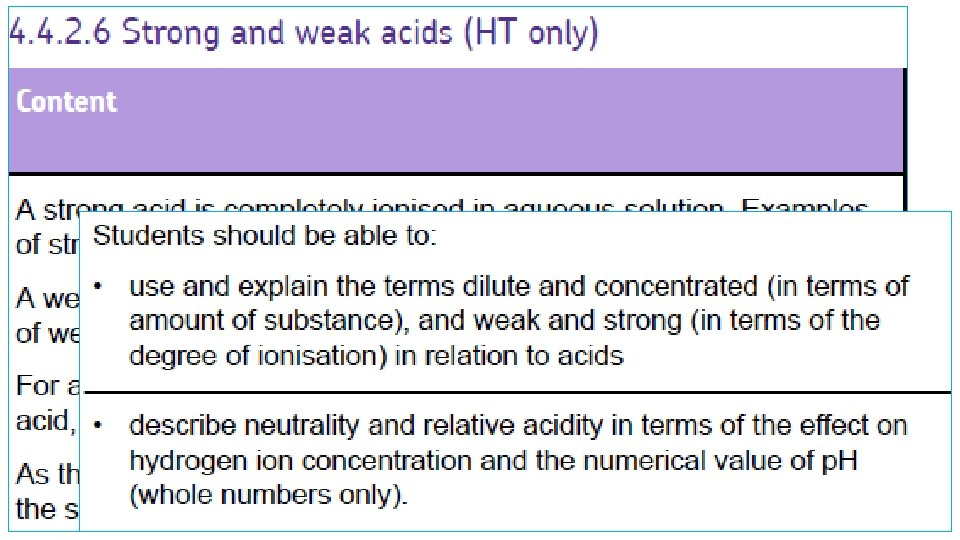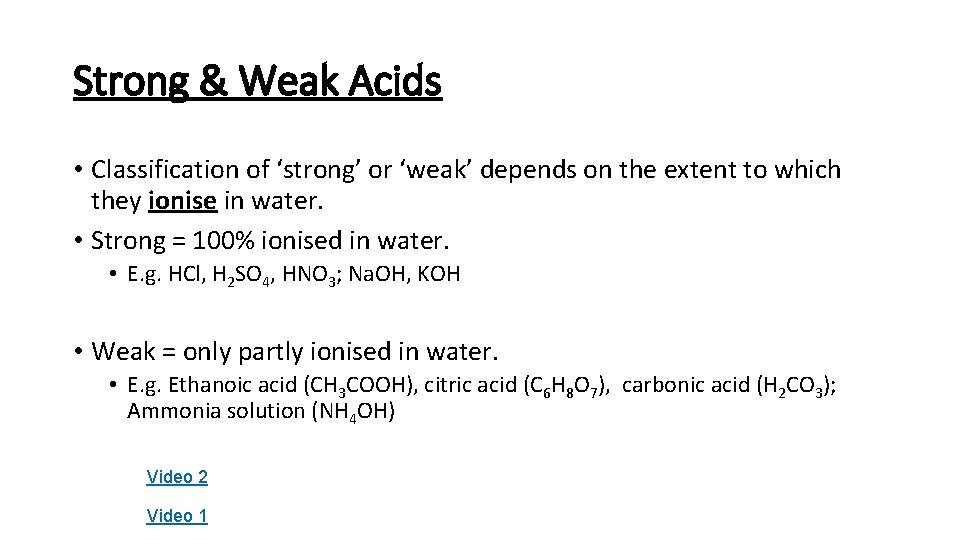Strong & Weak Acids • Classification of ‘strong’ or ‘weak’ depends on the extent to which they ionise in water. • Strong = 100% ionised in water. • E. g. HCl, H 2 SO 4, HNO 3; Na. OH, KOH • Weak = only partly ionised in water. • E. g. Ethanoic acid (CH 3 COOH), citric acid (C 6 H 8 O 7), carbonic acid (H 2 CO 3); Ammonia solution (NH 4 OH) Video 2 Video 1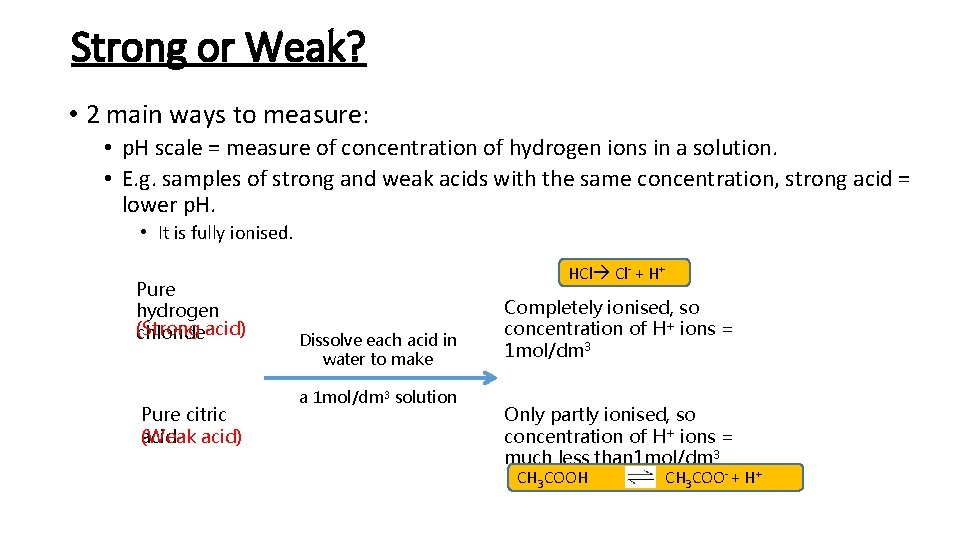Strong or Weak? • 2 main ways to measure: • p. H scale = measure of concentration of hydrogen ions in a solution. • E. g. samples of strong and weak acids with the same concentration, strong acid = lower p. H. • It is fully ionised. Pure hydrogen (Strong chlorideacid) Pure citric acid) (Weak HCl Cl- + H+ Dissolve each acid in water to make a 1 mol/dm 3 solution Completely ionised, so concentration of H+ ions = 1 mol/dm 3 Only partly ionised, so concentration of H+ ions = much less than 1 mol/dm 3 CH 3 COOH CH 3 COO- + H+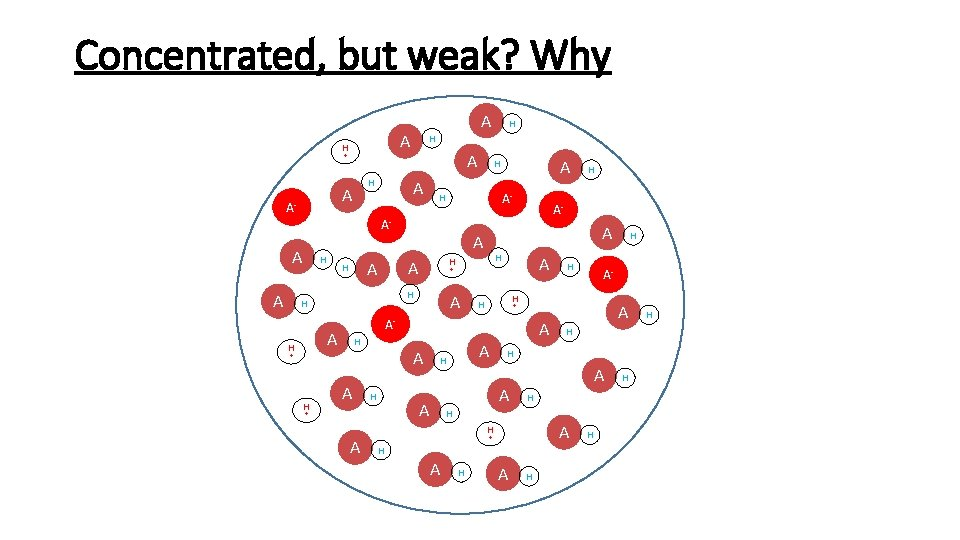Concentrated, but weak? Why A H + H A A- A H A A A H H A- H H H A + A + H A A H H A H A + H A H H A- + A A + H H H A- A- A H A H H H H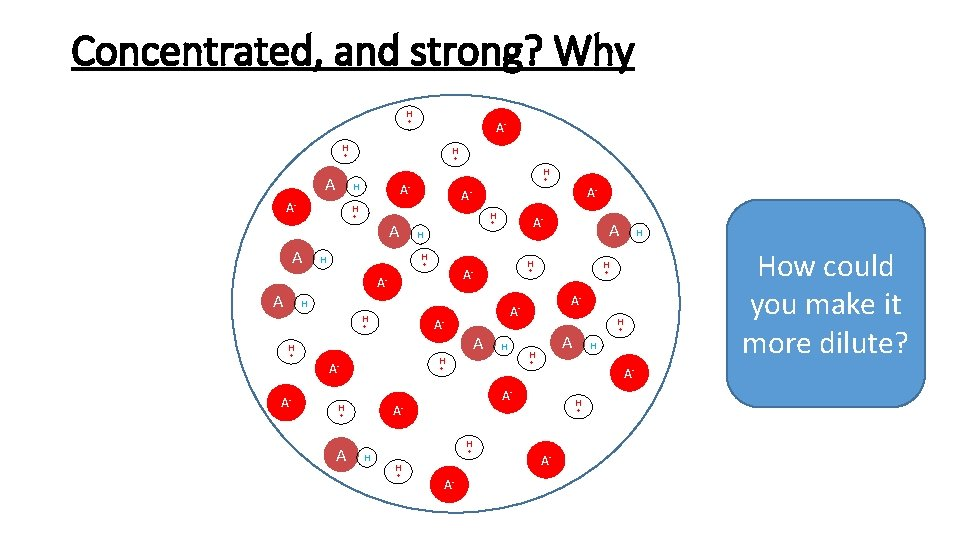Concentrated, and strong? Why H + A- H H + + H A- + A- H H + A A A- + H H H + AA- H H + + H A- H H + A H A- A- + + H A H H A- How could you make it more dilute? + A- A- + H H A H A- A- A-Strong or Weak? • Or – by observing the rate of reaction, when a reactive metal is added.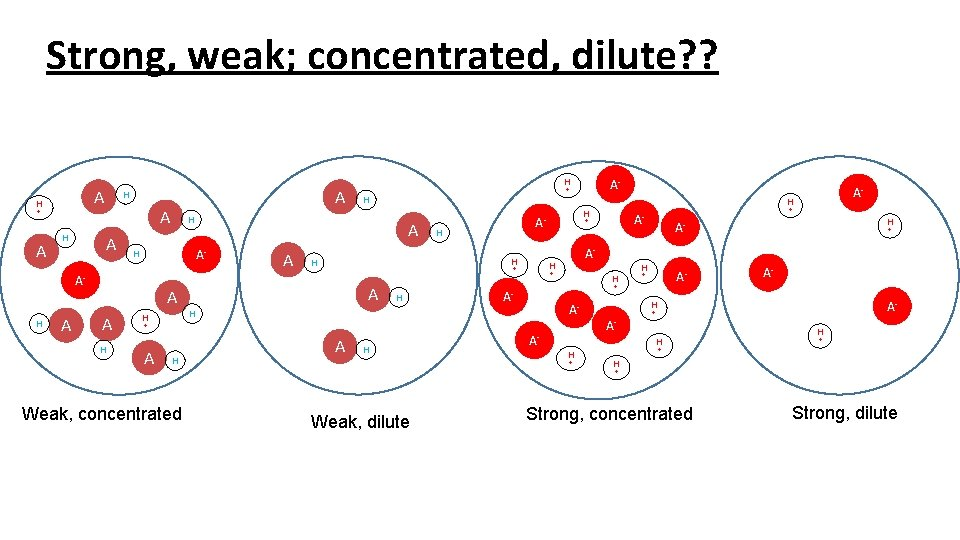Strong, weak; concentrated, dilute? ? A H + A H A A A H H A H A- + A H Weak, concentrated A- H A- + + + AA- H + AH H H A- + A- H H + A- + H + A- H H A A- + A A- A A H H H AH A H H + + H + Weak, dilute Strong, concentrated Strong, dilute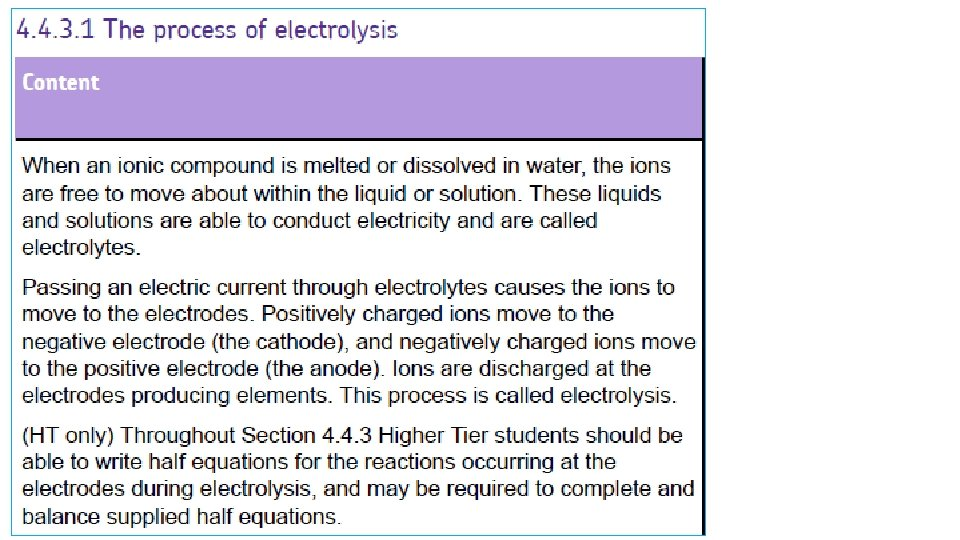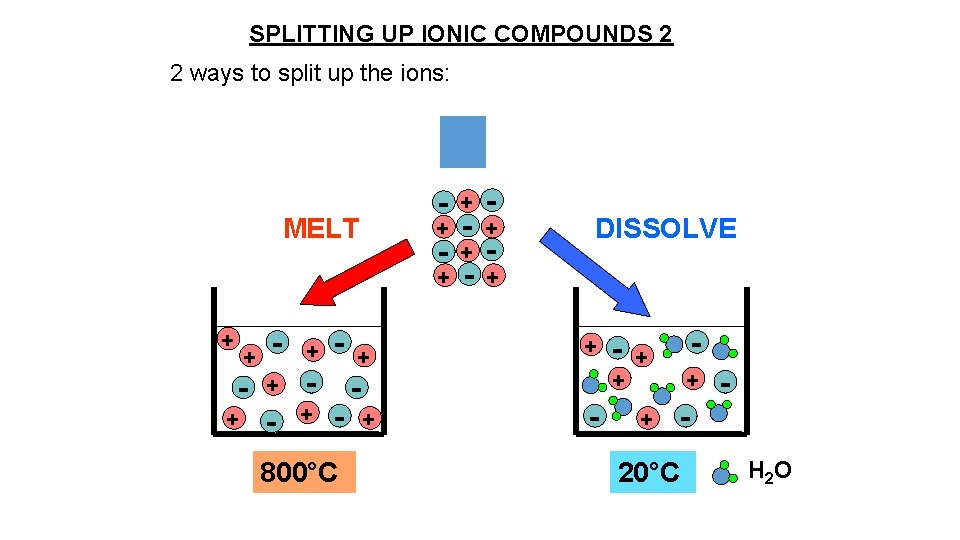SPLITTING UP IONIC COMPOUNDS 2 2 ways to split up the ions: MELT + + - + - + 800°C - + + - - + - DISSOLVE + - + + + - - - + 20°C H 2 O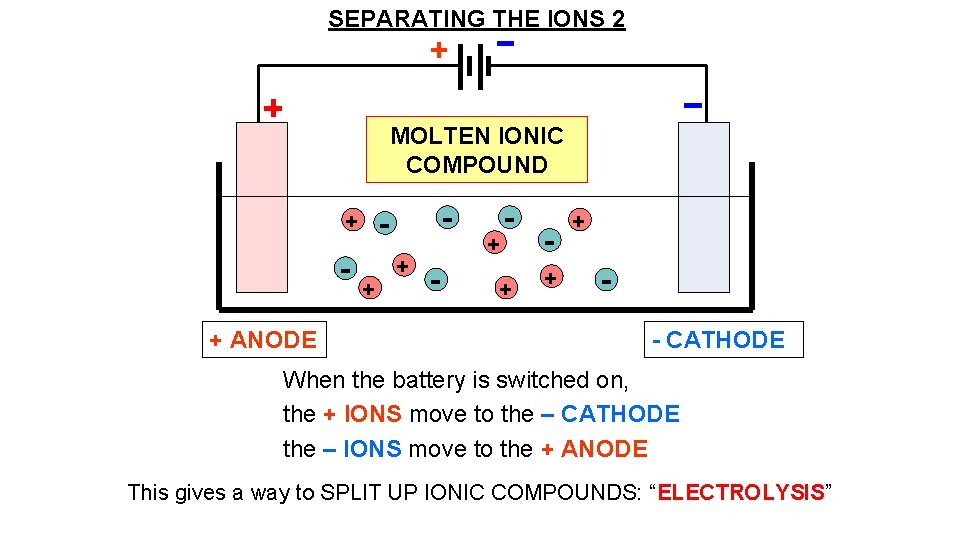SEPARATING THE IONS 2 + MOLTEN IONIC COMPOUND - + ANODE + + - + + + - CATHODE When the battery is switched on, the + IONS move to the – CATHODE the – IONS move to the + ANODE This gives a way to SPLIT UP IONIC COMPOUNDS: “ELECTROLYSIS”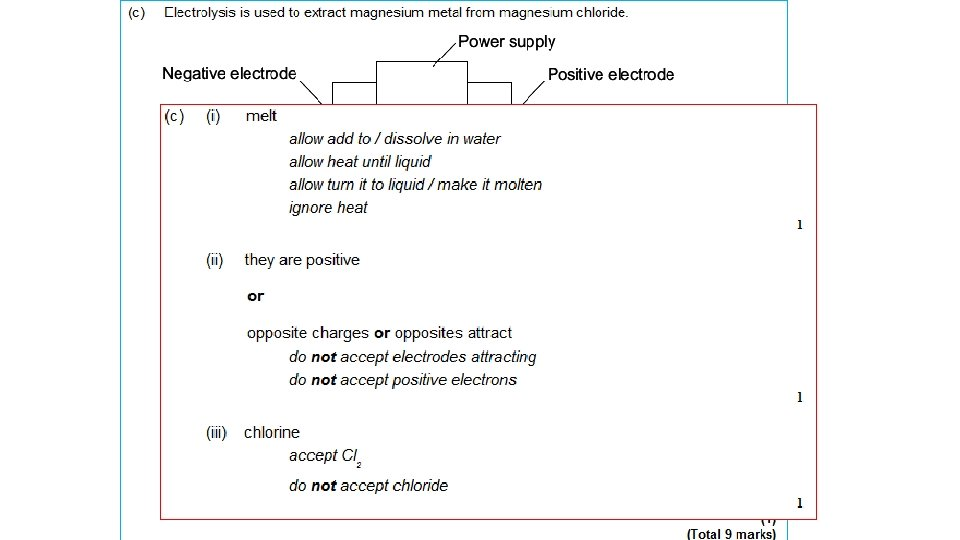Example 1: Splitting up MOLTEN SODIUM CHLORIDE (salt) - = Cl- chloride ION, extra 1 electron Cl chlorine ATOM, Cl Cl NEUTRAL Cl 2 molecule + Cl Cl Cl- - Cl Cl At ANODE: Clthen: Cl + Cl - chloride IONS lose their extra electrons and turn into neutral chlorine ATOMS e- + Cl Cl 2 (gas) Both together: 2 Cl- → 2 e- + Cl 2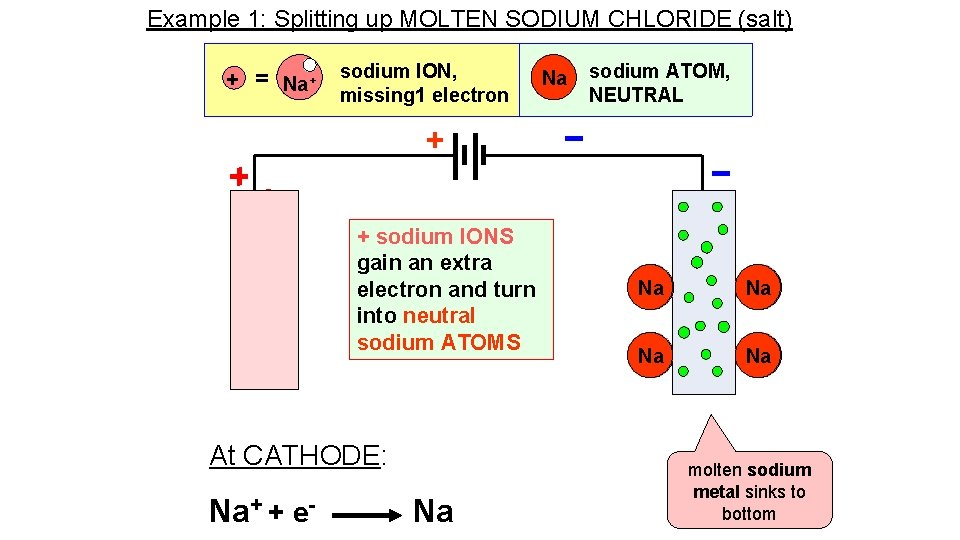Example 1: Splitting up MOLTEN SODIUM CHLORIDE (salt) + = Na+ sodium ION, missing 1 electron Na sodium ATOM, NEUTRAL + + sodium IONS gain an extra electron and turn into neutral sodium ATOMS At CATHODE: Na+ + e- Na Na+ Na molten sodium metal sinks to bottom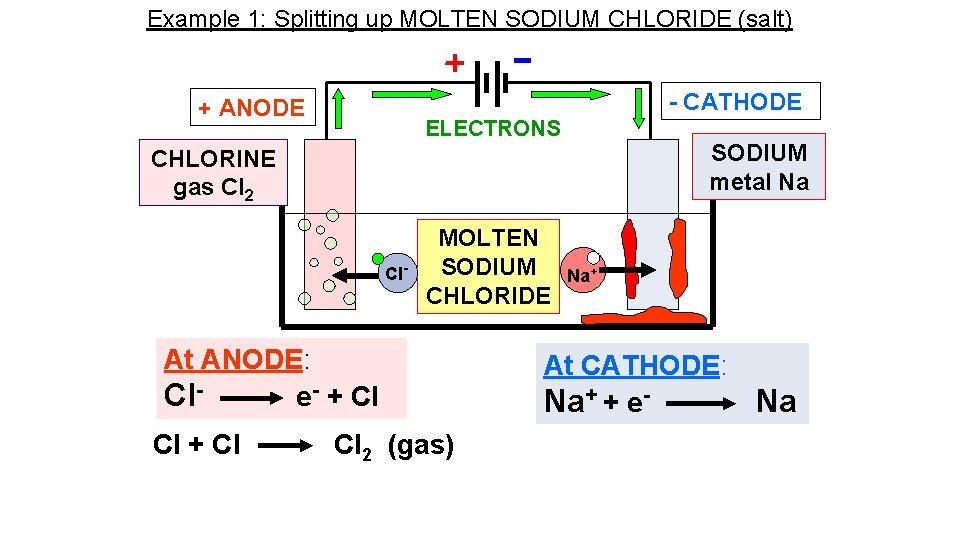Example 1: Splitting up MOLTEN SODIUM CHLORIDE (salt) - CATHODE + ANODE ELECTRONS SODIUM metal Na CHLORINE gas Cl 2 Cl- MOLTEN SODIUM CHLORIDE At ANODE: Cle- + Cl Cl 2 (gas) Na+ At CATHODE: Na+ + e- Na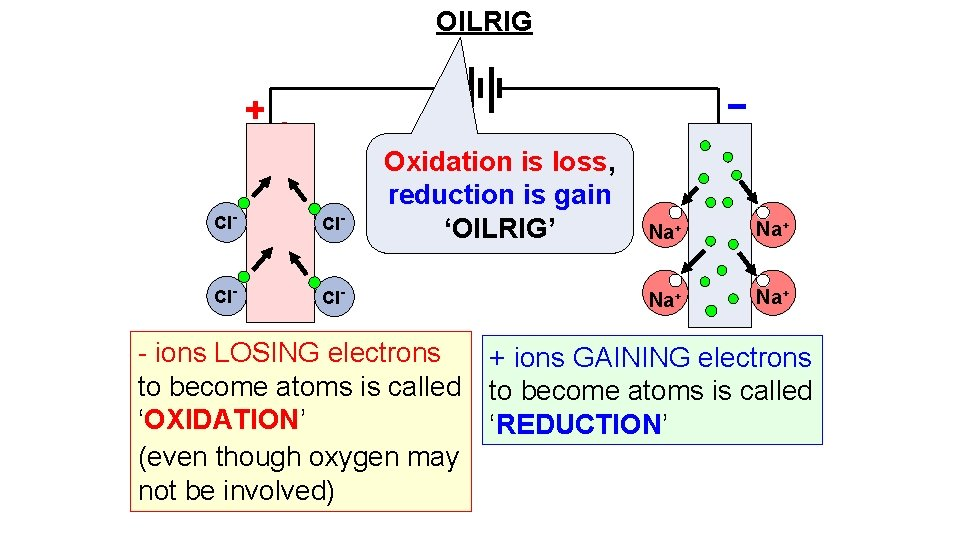OILRIG Cl- Cl- Oxidation is loss, reduction is gain ‘OILRIG’ Na+ Na+ - ions LOSING electrons + ions GAINING electrons to become atoms is called ‘OXIDATION’ ‘REDUCTION’ (even though oxygen may not be involved)Example 2: Splitting up MOLTEN LEAD BROMIDE Pb. Br 2 - CATHODE + ANODE ELECTRONS LEAD Metal Pb BROMINE gas Br 2 Br- MOLTEN LEAD BROMIDE At ANODE: Bre- + Br Br 2 (gas) Pb 2+ At CATHODE: Pb 2+ + 2 e- Both together: 2 Br- → 2 e- + Br 2 Pb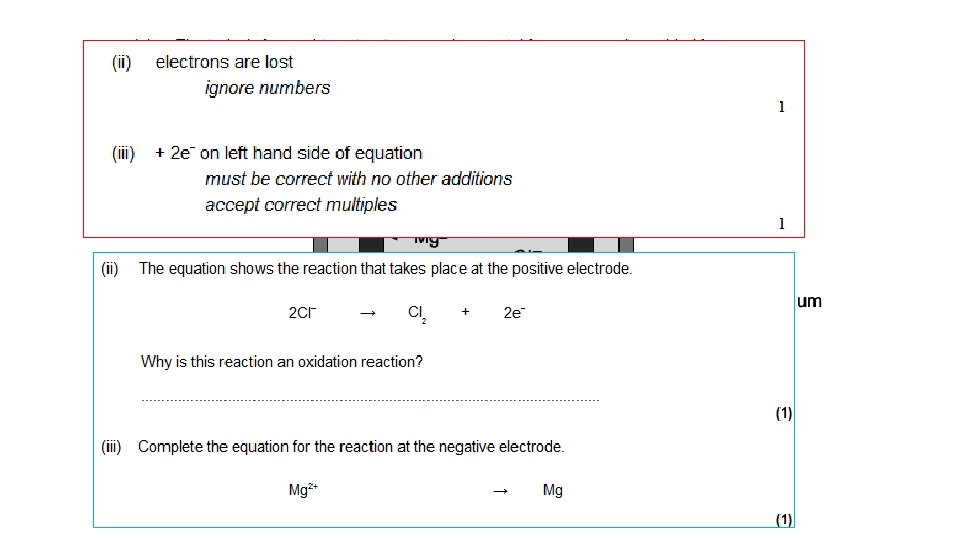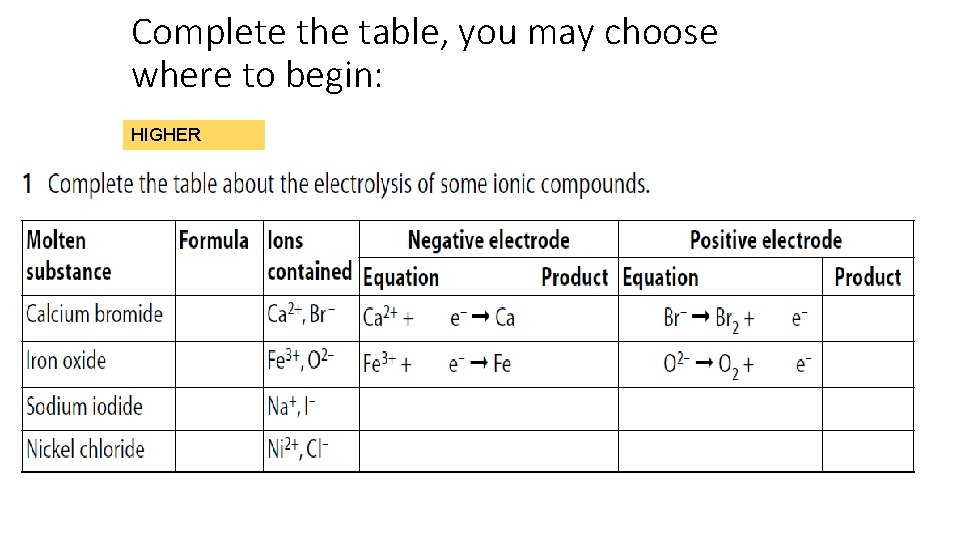Complete the table, you may choose where to begin: HIGHER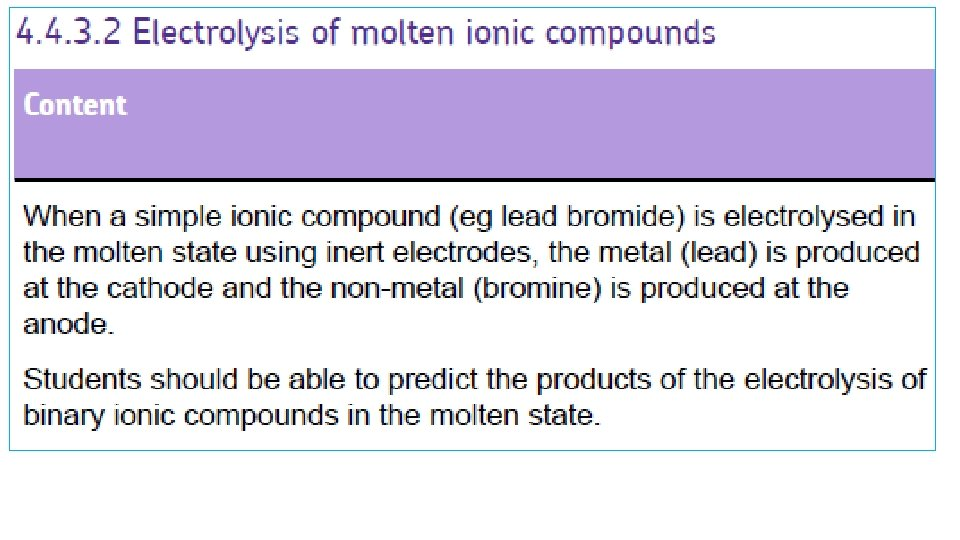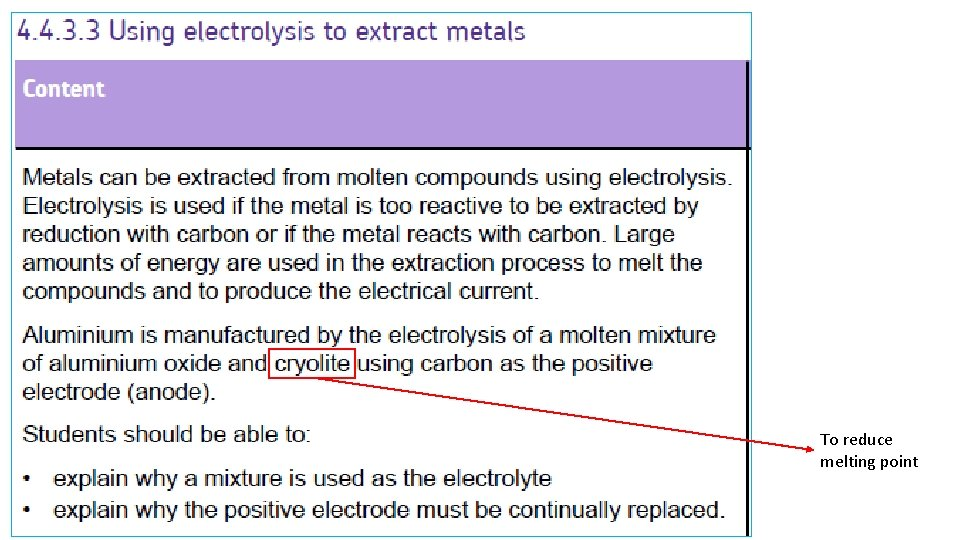To reduce melting point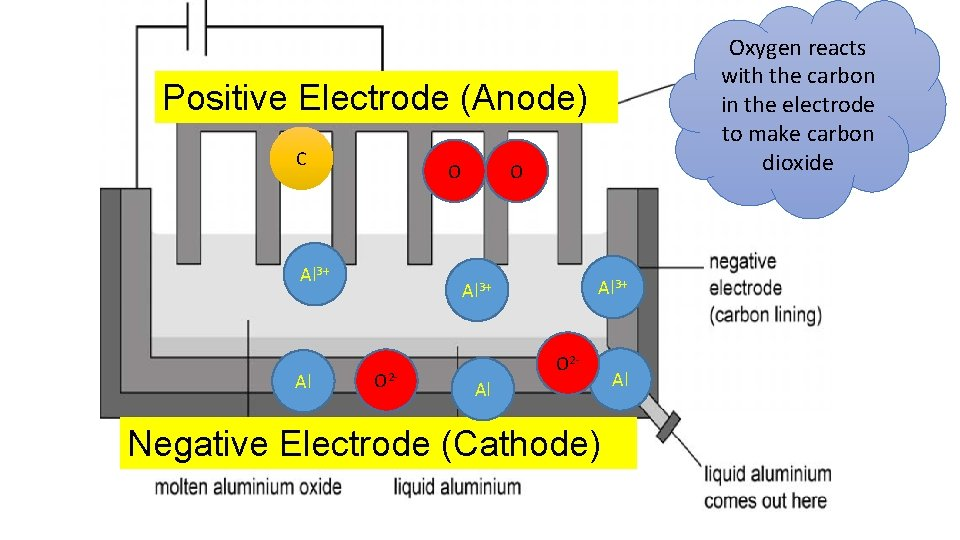Oxygen reacts with the carbon in the electrode to make carbon dioxide Positive Electrode (Anode) C O Al 3+ O 2 - O 2 Al Negative Electrode (Cathode) Al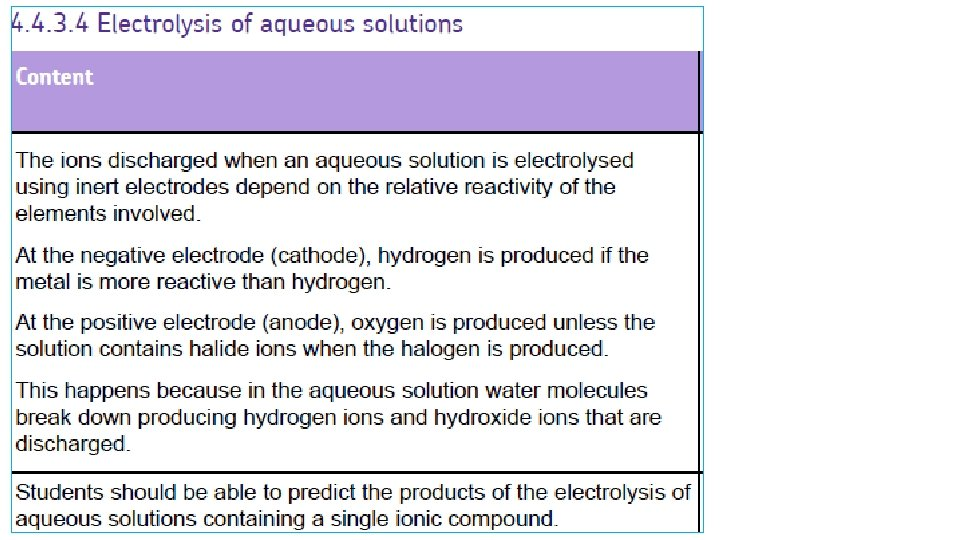What happens when the ionic compounds are dissolved in water? Here, water molecules break up into HYDROGEN IONS, H+ and HYDROXIDE IONS OH- H 2 O H+ + OHSo, in an ionic solution (eg sodium chloride solution), there will be FOUR types of ion present: TWO from the ionic compound and TWO from the water (H+ SODIUM CHLORIDE SOLUTION Na. Cl (aq) Cl- H+ OH- Na+ OHH+ Na+ Cl- H+ OH- Na+ Cl- + OH-)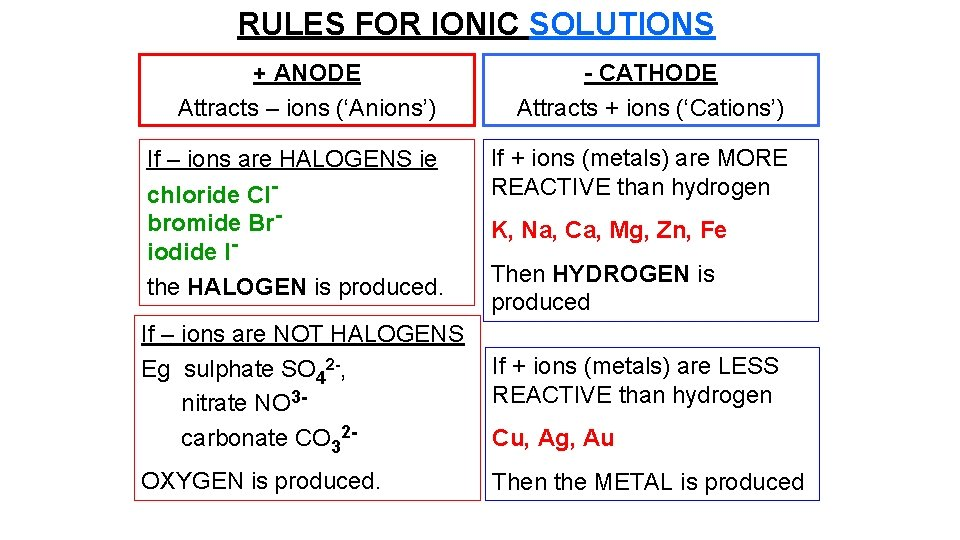RULES FOR IONIC SOLUTIONS + ANODE Attracts – ions (‘Anions’) If – ions are HALOGENS ie chloride Clbromide Briodide Ithe HALOGEN is produced. - CATHODE Attracts + ions (‘Cations’) If + ions (metals) are MORE REACTIVE than hydrogen K, Na, Ca, Mg, Zn, Fe Then HYDROGEN is produced If – ions are NOT HALOGENS Eg sulphate SO 42 -, nitrate NO 3 carbonate CO 32 - If + ions (metals) are LESS REACTIVE than hydrogen OXYGEN is produced. Then the METAL is produced Cu, Ag, Au(REACTIVITY: K+ Na+ Ca 2+ Mg 2+ Al 3+ Zn 2+ Fe 3+ H+ Cu 2+ Ag+ Au 3+ ) Compound State Ions Cathode (-) Anode (+) potassium molten chloride aluminium molten oxide copper chloride solution sodium bromide silver nitrate solution potassium chloride zinc sulphate solution K+ Cl- potassiu m chlorine solution (REACTIVITY: K+ Na+ Ca 2+ Mg 2+ Al 3+ Zn 2+ Fe 3+ H+ Cu 2+ + 3+